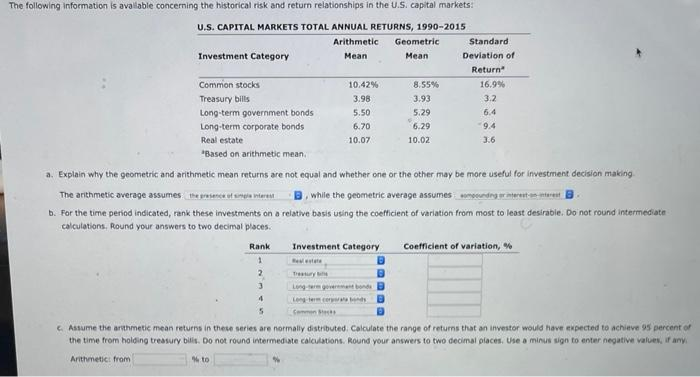Home / Expert Answers / Accounting / a-explain-why-the-geometric-and-arithmetic-mean-returns-are-not-equal-and-whether-one-or-the-other-pa519

# (Solved): a. Explain why the geometric and arithmetic mean returns are not equal and whether one or the other ...a. Explain why the geometric and arithmetic mean returns are not equal and whether one or the other may be more useful for investment decision making The arithmetic average assumes: While the geometric average assumes b. For the time period indicated, rank these investments on a relative basis using the coefficient of variation from most to least desirable. Do not round intermediate calculations, Round your answers to two decimal plsces. C. Assume the arahmetic mean returns in these series are normaliy distributed. Calculate the range of retums that an investor would have eipected to achieve 95 percent of the time from holding treasury bilis. Do not round intermeduate calculations. Round your answers to two decimal glaces. Use a minus sign fo enter negative values, if anyi Arithmetici from

We have an Answer from Expert